# Free Two Digit Addition Worksheets

###Adding Tens to a Number

Common Core State Standards: 2.NBT.5 Fluently add and subtract within 100....
Add tens to numbers. Includes a hundred chart.

###Adding Tens Together

Common Core State Standards: 2.NBT.5 Fluently add and subtract within 100....

###Counting on Tens and Ones

Common Core State Standards: 2.NBT.5 Fluently add and subtract within 100....
Counting on ones and tens. Includes a hundred chart.

###Rounding to Find the Sums2,  3

Common Core State Standards: 2.NBT.5 Fluently add and subtract within 100....
Round 2 two-digit numbers to find the sums.

###Adding Two Digit and One Digit Numbers2,  3

Common Core State Standards: 2.NBT.5 Fluently add and subtract within 100....
Add two digit and one digit numbers worksheet. 25 problems.

###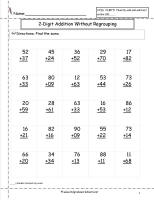Two Digit Addition With No Regrouping2

Common Core State Standards: 2.NBT.5 Fluently add and subtract within 100....
Two digit addition with no regrouping worksheet.  25 problems.

###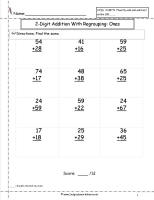Two Digit Addition With Regrouping Ones to Tens 2, 3, 4, 5, 6, 7, 8, 9, 10

Common Core State Standards: 2.NBT.5 Fluently add and subtract within 100....
Two digit addition with regrouping ones to tens place worksheet.  12 problems.

###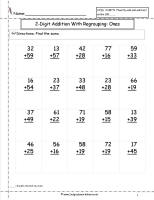Two Digit Addition With Regrouping Ones to Tens 2, 3,   4, 5

Common Core State Standards: 2.NBT.5 Fluently add and subtract within 100....
Two digit addition with regrouping ones to tens place worksheet.  20 problems.

###Two Digit Addition With Regrouping Ones to Tens 2,3, 4, 5

Common Core State Standards: 2.NBT.5 Fluently add and subtract within 100....
Two digit addition with regrouping ones to tens place worksheet.  25 problems.

###Two Digit Addition With Regrouping Ones to Tens Baseline Assessment2,3

Common Core State Standards: 2.NBT.5 Fluently add and subtract within 100....
Two digit addition with regrouping ones to tens place worksheet baseline assessment worksheet.  20 problems.

###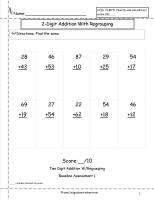Two Digit Addition With Regrouping Ones to Tens Baseline Assessment 2, 3

Common Core State Standards: 2.NBT.5 Fluently add and subtract within 100....
Two digit addition with regrouping ones to tens place worksheet baseline assessment worksheet.  10 problems.

###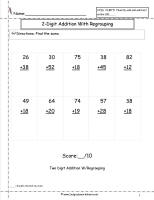Two Digit Addition With Regrouping Ones to Tens  Assessment2,3

Common Core State Standards: 2.NBT.5 Fluently add and subtract within 100....
Two digit addition with regrouping ones to tens place worksheet assessment worksheet.  10 problems.

###Two Digit Addition With Regrouping Ones to Tens Screening Assessment2,3

Common Core State Standards: 2.NBT.5 Fluently add and subtract within 100....
Two digit addition with regrouping ones to tens place worksheet baseline assessment worksheet.  20 problems.

###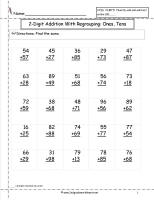Two Digit Addition With Regrouping Ones and Tens Place

Common Core State Standards: 2.NBT.5 Fluently add and subtract within 100....
Two digit addition with regrouping ones and tens place worksheet.  20 problems.

###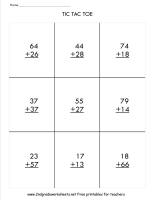Two Digit Addition With Regrouping Tic Tac Toe Game

Common Core State Standards: 2.NBT.5 Fluently add and subtract within 100....
Two digit addition with regrouping ones to tens place Tic Tac Toe game.  10 games.

###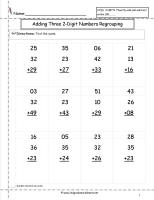Two Digit Addition With Regrouping-Three Numbers

Common Core State Standards: 2.NBT.5 Fluently add and subtract within 100....
Three number-two digit addition with regrouping ones to tens.